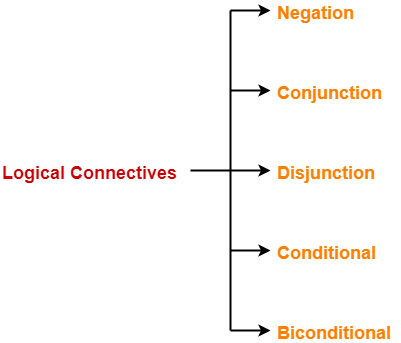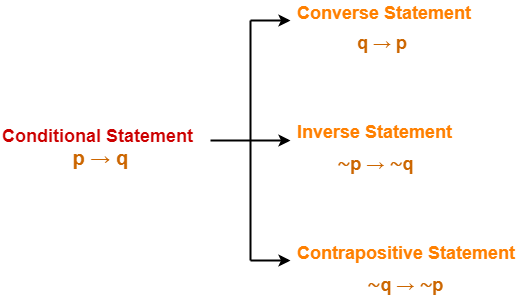# Converse Inverse Contrapositive | Problems

## Logical Connectives-

Before you go through this article, make sure that you have gone through the previous article on Logical Connectives.

We have discussed-

• Logical connectives are the operators used to combine one or more propositions.
• In propositional logic. there are 5 basic connectives-In this article, we will discuss Converse, Inverse and Contrapositive of a conditional statement.

## Converse, Inverse and Contrapositive-

For a conditional statement p → q,

• The converse statement is q → p
• The inverse statement ∼p → ∼q
• The contrapositive statement is ∼q → ∼p## Note-01:

• For conditional statements (p → q) only, the converse, inverse and contrapositive statements can be written.

## Note-02:

 Performing any two actions always result in the third one.

For example-

• Inverse of converse is contrapositive.
• Inverse of contrapositive is converse.
• Converse of inverse is contrapositive.
• Converse of contrapositive is inverse.
• Contrapositive of inverse is converse.
• Contrapositive of converse is inverse.

## Note-03:

For a conditional statement p → q,

• Its converse statement (q → p) and inverse statement (∼p → ∼q) are equivalent to each other.
• p → q and its contrapositive statement (∼q → ∼p) are equivalent to each other.

## Problem-01:

Write the converse, inverse and contrapositive of the following statements-

1. If today is Sunday, then it is a holiday.
2. If 5x – 1 = 9, then x = 2.
3. If it rains, then I will stay at home.
4. I will dance only if you sing.
5. I will go if he stays.
6. We leave whenever he comes.
7. You will qualify GATE only if you work hard.
8. If you are intelligent, then you will pass the exam.

## Solution-

### Part-01:

We have-

• The given sentence is- “If today is Sunday, then it is a holiday.”
• This sentence is of the form- “If p then q”.

So, the symbolic form is p → q where-

p : Today is Sunday

q : It is a holiday

Converse Statement- If it is a holiday, then today is Sunday.

Inverse Statement- If today is not Sunday, then it is not a holiday.

Contrapositive Statement- If it is not a holiday, then today is not Sunday.

### Part-02:

We have-

• The given sentence is- “If 5x – 1 = 9, then x = 2.”
• This sentence is of the form- “If p then q”.

So, the symbolic form is p → q where-

p : 5x – 1 = 9

q : x = 2

Converse Statement- If x = 2, then 5x – 1 = 9.

Inverse Statement- If 5x – 1 ≠ 9, then x ≠ 2.

Contrapositive Statement- If x ≠ 2, then 5x – 1 ≠ 9.

### Part-03:

We have-

• The given sentence is- “If it rains, then I will stay at home.”
• This sentence is of the form- “If p then q”.

So, the symbolic form is p → q where-

p : It rains

q : I will stay at home

Converse Statement- If I will stay at home, then it rains.

Inverse Statement- If it does not rain, then I will not stay at home.

Contrapositive Statement- If I will not stay at home, then it does not rain.

### Part-04:

We have-

• The given sentence is- “I will dance only if you sing.”
• This sentence is of the form- “p only if q”.

So, the symbolic form is p → q where-

p : I will dance

q : You sing

Converse Statement- If you sing, then I will dance.

Inverse Statement- If I will not dance, then you do not sing.

Contrapositive Statement- If you do not sing, then I will not dance.

### Part-05:

We have-

• The given sentence is- “I will go if he stays.”
• This sentence is of the form- “q if p”.

So, the symbolic form is p → q where-

p : He stays

q : I will go

Converse Statement- If I will go, then he stays.

Inverse Statement- If he does not stay, then I will not go.

Contrapositive Statement- If I will not go, then he does not stay.

### Part-06:

We have-

• The given sentence is- “We leave whenever he comes.”
• We can replace “whenever” with “if”.
• Then, the sentence is- “We leave if he comes.”
• This sentence is of the form- “q if p”.

So, the symbolic form is p → q where-

p : He comes

q : We leave

Converse Statement- If we leave, then he comes.

Inverse Statement- If he does not come, then we do not leave.

Contrapositive Statement- If we do not leave, then he does not come.

### Part-07:

We have-

• The given sentence is- “You will qualify GATE only if you work hard.”
• This sentence is of the form- “p only if q”.

So, the symbolic form is p → q where-

p : You will qualify GATE

q : You work hard

Converse Statement- If you work hard, then you will qualify GATE.

Inverse Statement- If you will not qualify GATE, then you do not work hard.

Contrapositive Statement- If you do not work hard, then you will not qualify GATE.

### Part-08:

We have-

• The given sentence is- “If you are intelligent, then you will pass the exam.”
• This sentence is of the form- “If p then q”.

So, the symbolic form is p → q where-

p : You are intelligent

q : You will pass the exam

Converse Statement- If you will pass the exam, then you are intelligent.

Inverse Statement- If you are not intelligent, then you will not pass the exam.

Contrapositive Statement- If you will not pass the exam, then you are not intelligent.

## Problem-02:

What is the converse of the statement- “I stay only if you go”?

1. I stay if you go.
2. If I stay, then you go.
3. If you do not go, then I do not stay.
4. If I do not stay, then you go.

## Solution-

• Try solving this problem yourself.
• Solution is in the linked video lecture.
• Option (A) is correct.

To gain better understanding about converting Converse, Inverse and Contrapositive,

Watch this Video Lecture

Next Article- Tautology, Contradiction and Contingency

Get more notes and other study material of Propositional Logic.

SummaryArticle Name
Converse Inverse Contrapositive | Problems
Description
Converse Inverse Contrapositive- For a statement p → q, q → p is a converse statement, ∼p → ∼q is a inverse statement, ∼q → ∼p is contrapositive statement. Problems based on Converse, Inverse and Contrapositive.
Author
Publisher Name
Gate Vidyalay
Publisher Logo